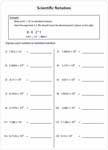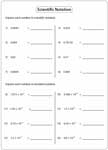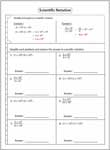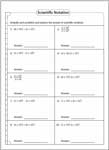1. Math Worksheets>
2. Pre-Algebra>
3. Scientific Notation

# Scientific Notation Worksheets

Scientific notation is a smart way of writing huge whole numbers and too small decimal numbers. This page contains worksheets based on rewriting whole numbers or decimals in scientific notation and rewriting scientific notation form to standard form. This set of printable worksheets is specially designed for students of grade 6, grade 7, grade 8, and high school. Access some of them for free!

## Scientific Notation - Positive Exponents

Express in Scientific Notation

Each pdf worksheet contains 14 problems rewriting whole numbers to scientific notation. Easy level has whole numbers up to 5-digits; Moderate level has more than 5-digit numbers.Easy:

Moderate:

Express in Standard Notation

Students of 6th grade need to express each scientific notation in standard notation. An example is provided in each worksheet.Easy:

Moderate:

Both Standard and Scientific Notations:

Each printable worksheet contains expressing numbers in both scientific and standard form.Easy:

Moderate:

## Scientific Notation - Negative Exponents

Rewrite in Scientific Notation

Rewrite the given decimals in scientific notation. Move the decimal point to the left until you get the first non-zero digit. The number of steps you moved represent the power (index) of 10.Easy:

Moderate:

Rewrite in Standard Notation

In this set of pdf worksheets, express each number in standard notation. Easy level has indices more than -5; Moderate level has indices less than -4.Easy:

Moderate:

Both Standard and Scientific Notations:

Each worksheet has ten problems expressing decimals in both standard and scientific notation.Easy:

Moderate:

## Scientific Notation - Mixed Exponents

Convert to Scientific Notation

The printable worksheets in this section contain expressing both whole numbers and decimals in scientific notation.Easy:

Moderate:

Convert to Standard Notation

The exponent in each scientific notation can be either positive or negative.Easy:

Moderate:

Both Standard and Scientific Notations:

This section of pdf worksheets gives the complete review in rewriting numbers in both standard and scientific notation. Both positive and negative exponents included.Easy:

Moderate:

## Scientific Notation - Math Operations

This section reinforces the knowledge in adding and subtracting numbers in scientific notation.Easy:

Moderate:

Difficult:

Multiplication and Division

Use laws of exponents (indices) to multiply and divide the expressions. Express the final answer in scientific notation.Easy:

Moderate:

Difficult:

Simplify the Expression

Each worksheet gives the complete review in performing operations with scientific notations, making it ideal for 7th grade, 8th grade, and high school students.Easy:

Moderate:

Difficult:

Related Worksheets